# Congruence of Triangles Class 9 Similarity Rules and formulas

CBSE Maths Congruence Of Triangles Class 9 Similarity Rules And Formulas

Chapter 7 CBSE Maths Congruence of Triangles Class 9 Similarity Rules and formulas

## Congruence of Triangles Class 9 similarly

##### Triangle

A closed figure formed by three intersecting lines is called a triangle. A triangle has three sides, three angles and three vertices.

##### Congruent figures

Congruent means equal in all respects or figures whose shapes and sizes are both the same for example, two circles of the same radii are congruent. Also two squares of the same sides are congruent.

##### Congruent Triangles

two triangles are congruent if and only if one of them can be made to superpose on the other, so as to cover it exactly.

• If two triangles ABC and PQR are congruent under the correspondence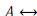P,B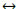Q and then symbolically, it is expressed as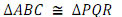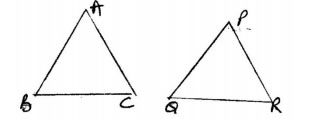• In congruent triangles corresponding parts are equal and we write 'CPCT' for corresponding parts of congruent triangles.

##### SAS congruency rule

Two triangles are congruent if two sides and the included angle of one triangle are equal to the two sides and the included angle of the other triangle.
For example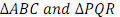as shown in the figure satisfy SAS congruent criterion.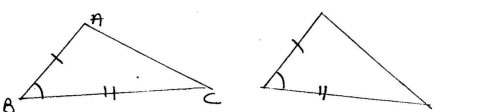##### ASA Congruence Rule -

Two triangles are congruent if two angles and the included side of one triangle are equal to two angles and the included side of other triangle.
. For examples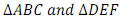shown below satisfy ASA congruence criterion.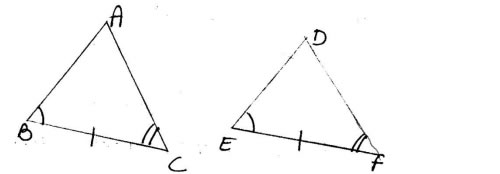##### AAS Congruence Rule

Two triangle are congruent if any two pairs of angles and one pair of corresponding sides are equal
for example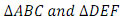shown below satisfy AAS congruence criterion.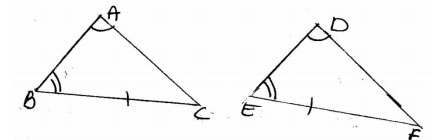• AAS criterion for congruence of triangles is a particular case of ASA criterion.

##### Isosceles Triangle

A triangle in which two sides are equal is called an isosceles triangle. For example shown below is an isosceles triangle with AB=AC.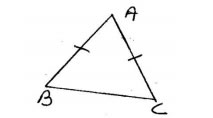• Angle opposite to equal sides of a triangle are equal.

• Sides opposite to equal angles of a triangle are equal.

• Each angle of an equilateral triangle is 60o .

##### SSS congruence Rule

If three sides of one triangle are equal to the three sides of another triangle then the two triangles are congruent.
For example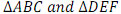as shown in the figure satisfy SSS congruence criterion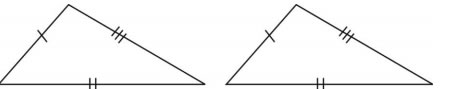##### RHS Congruence Rule

- If in two right triangles the hypotenuse and one side of one triangle are equal to the hypotenuse and one side of the other triangle then the two triangle are congruent.
For example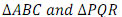shown below satisfy RHS congruence criterion.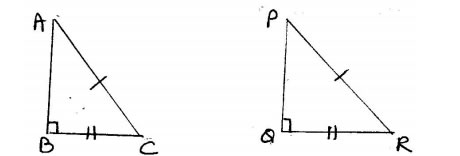##### RHS stands for right angle

Hypotenuse side.

• A point equidistant from two given points lies on the perpendicular bisector of the line segment joining the two points and its converse.

• A point equidistant from two intersecting lines lies on the bisectors of the angles formed by the two lines.

• In a triangle, angle opposite to the longer side is larger (greater)

• In a triangle, side opposite to the large (greater) angle is longer.

• Sum of any two sides of a triangle is greater than the third side.

Section - A

Q.1 Which of the following is not a criterion for congruence of triangles?
(a) SAS
(b) SSA
(c) ASA
(d) SSS

Q.2 If AB=QR, BC=PR and CA=PQ then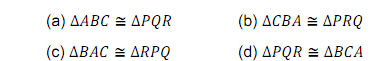Q.3 In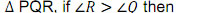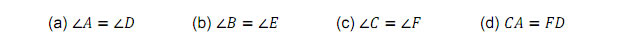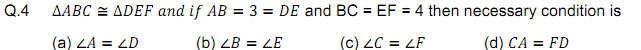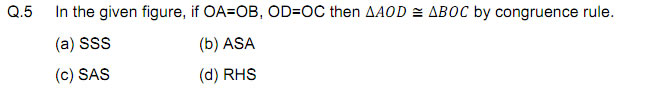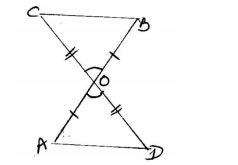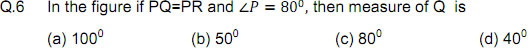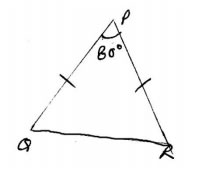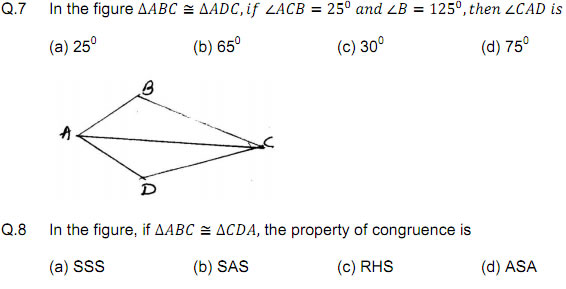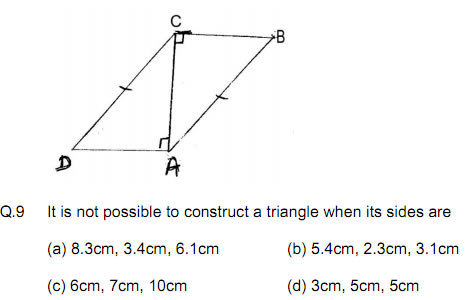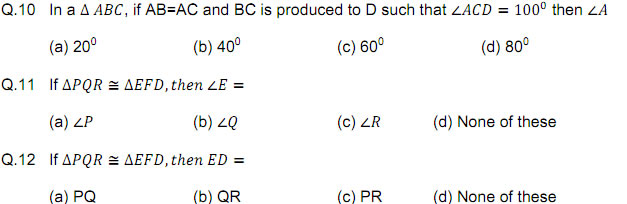Section - B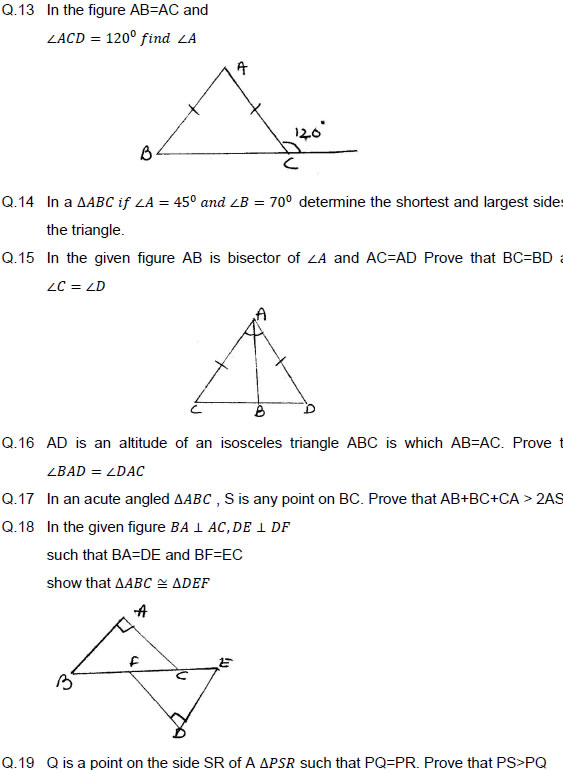Section - C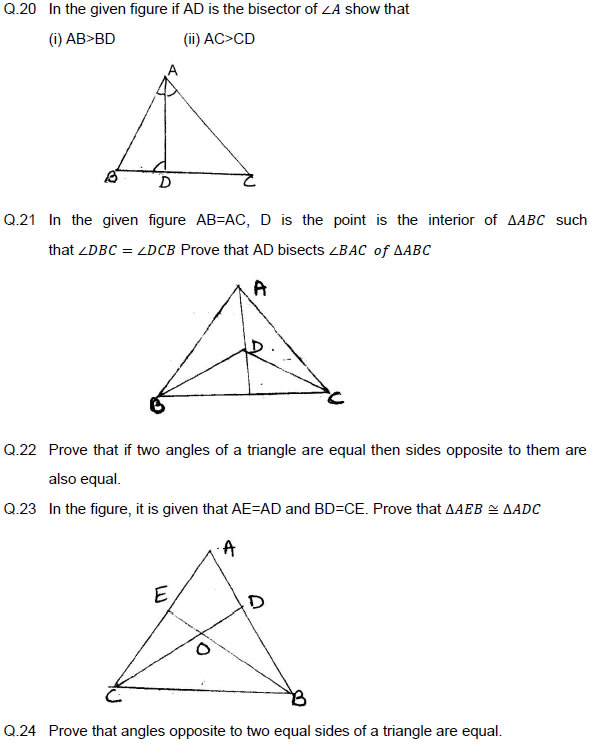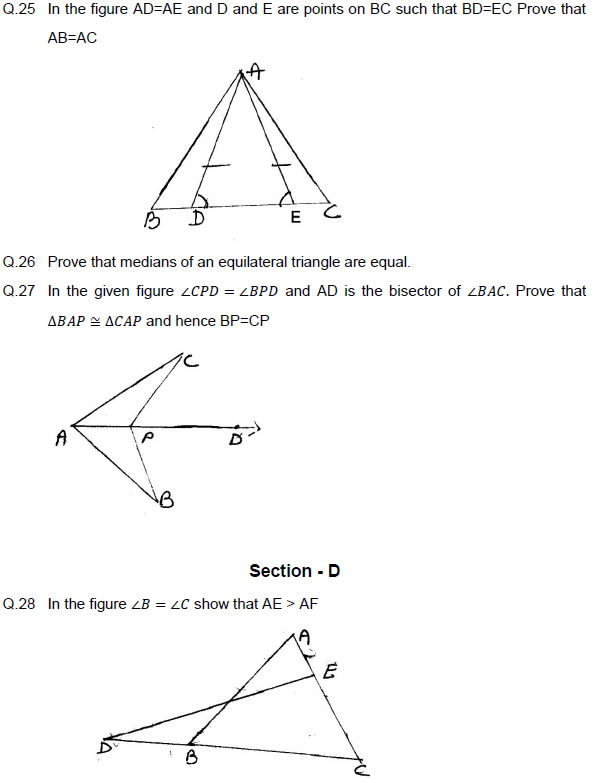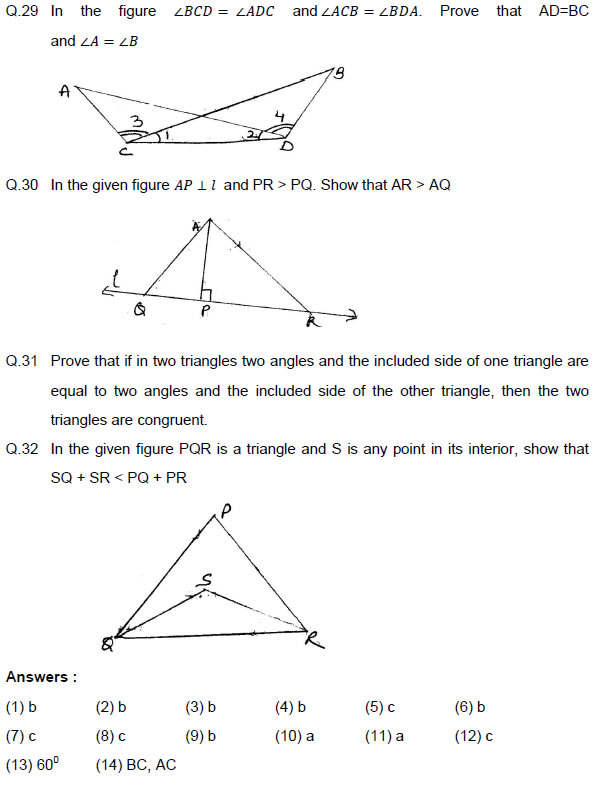### NCERT Books Free Pdf Download for Class 5, 6, 7, 8, 9, 10 , 11, 12 Hindi and English Medium

 Mathematics Biology Psychology Chemistry English Economics Sociology Hindi Business Studies Geography Science Political Science Statistics Physics Accountancy